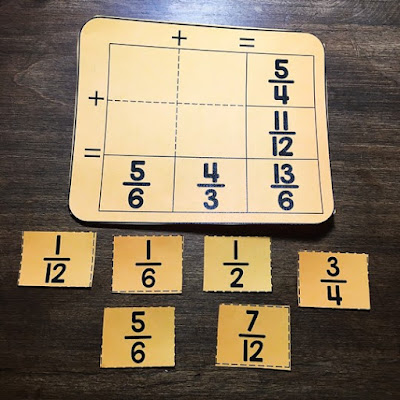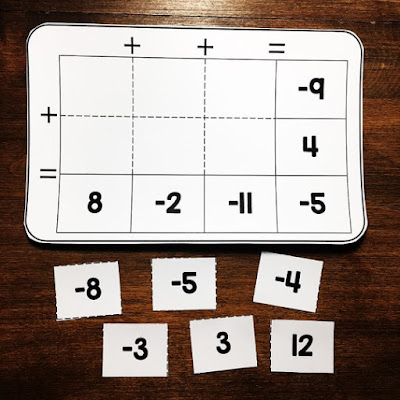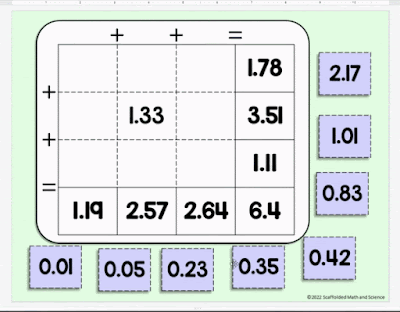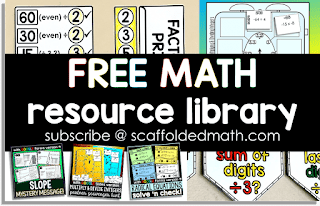# Print and Digital Math Puzzles

My daughter and I recently worked on a 550-piece puzzle, which took us just under a week to complete. We worked on it on the floor a bit each day after school, hiding it from the cats each night. It had been over 20 years since I had worked on a puzzle that was more than 30 pieces (kid puzzles), so it surprised me how much we both enjoyed it. Figuring out where the pieces went was relaxing and enjoyable, and I could feel it exercising my brain in ways that it doesn't usually exercise.

Working on that puzzle with my daughter got me thinking about making puzzles, so this past week I started making some math puzzle sets that cover various curriculum topics. Students can work on these math puzzles as classwork, in centers, with a partner or as a review activity.

### Print & Digital Math Puzzles

Each puzzle set comes with 3 puzzles: a 2x2 grid, a 2x3 grid, and a 3x3 grid with the middle number filled in. This way, you can easily differentiate for your students. Each set comes in printable PDF form and drag-and-drop digital in GOOGLE Slides within the same file.Adding fractions - puzzle #1 in the set

The first set coverers fraction addition. Above is puzzle #1 with all 6 sorting slips (including the 2 distractor answers). To lessen the difficulty of this puzzle, you can give students just the 4 slips that correctly complete the puzzle. Full answer keys are included to make this easy.Adding integers - puzzle #2 in the set

Next is a set of 3 puzzles covering integer addition. Above is puzzle #2 in the set with 6 blank spaces and 6 sorting slips. To lessen the intensity of this puzzle for students who need a bit more scaffolding, you can sort one of the integers onto the puzzle before giving the puzzle to students.Adding 2-digit decimals - puzzle #3 (digital version)

Above is what each puzzle's GOOGLE Slides version looks like. This is the GOOGLE Slides version of an adding 2-digit decimals puzzle (puzzle #3). Like puzzle #2 in each set, you can sort one or more of the sorting slips onto the puzzle before giving to students if you have students who need a little extra support.

Ideas for differentiating each puzzle set:

Puzzle #1 has 4 blank spaces and comes with 6 sorting numbers. Students figure out which numbers will add to the rows and columns. You can choose to give students all 6 sorting numbers with the puzzle to make the puzzle more challenging, or only the 4 sorting numbers that correctly complete the puzzle.

Puzzle #2 has 6 blank spaces and comes with 6 sorting numbers. To decrease the challenge of this puzzle, you can choose to sort one of the sorting numbers onto the puzzle before giving it to students.

Puzzle #3 has 8 blank spaces with 8 sorting numbers. It is a 3x3 grid with the middle number filled in. This will be the most challenging puzzle.

If you try any of these math puzzles with your students, I'd love to hear how it went!

> Browse all Math Puzzles.Free math resource library

Source: scaffoldedmath